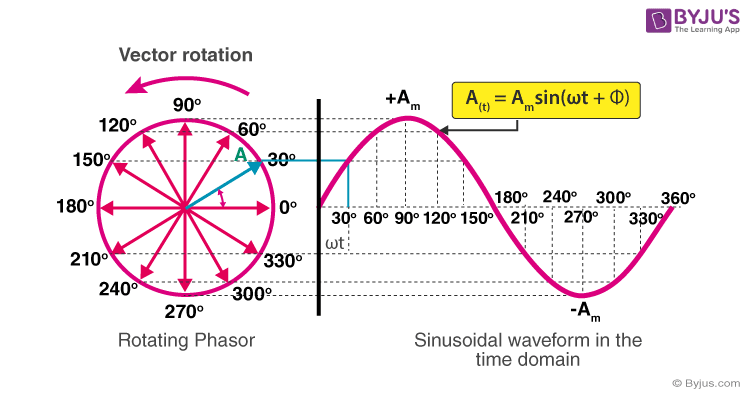Jet Set Go! All about Aeroplanes Jet Set Go! All about Aeroplanes

# Representation of AC current and voltage by phasor diagram

Alternating Current (AC) is a type of electric current that reverses its direction periodically in contrast to the Direct Current (DC) which flows in a single direction. We have read about electrical circuits which contain a resistor connected across an AC source, an inductor connected across an AC source, a capacitor connected across an AC source or the combination of any two or all three of these components connected across an AC source. We know that, in the device like a resistor, the current across the resistor is in phase with the voltage source. But for the devices like inductor or a capacitor, the current either lags or leads the voltage source to a certain value. This theory of phasors is used to relate the current and the voltage in the latter case. In this section, we will learn about the phasor diagrams in detail.A phasor is a vector that is used to represent a sinusoidal function. It rotates about the origin with an angular speed ω. The vertical component of phasors represents the quantities that are sinusoidally varying for a given equation, such as v and i. Here, the magnitude of the phasors represents the peak value of the voltage and the current. From the figure shown above, we can see the relation between a phasor and the sinusoidal representation of the function with respect to time. The projection of the phasor on the vertical axis represents the value of the quantity. For example, in the case of a current or a vector phasor, the projection of the phasor on the vertical axis, given by vmsinωt and imsinωt respectively, gives the value of the current or the voltage at that instant. From the phasor diagram, it is easy to detect that one of two quantities are in the same phase.

For example, if for a given circuit, the phasors for the voltage and the current are in the same direction for all instances, the phase angle between the voltage and the current is zero.

The video examines the questions asked on the topic of alternating current by analyzing previous year’s JEE Mains & Advanced papers.## Frequently Asked Questions – FAQs

### What is an inductor?

It is a two-terminal passive electrical component that opposes sudden changes in current. It is used to store energy in the form of magnetic energy when electricity is applied to it.

### What is a capacitor?

A capacitor is a two-terminal electrical device that can store energy in the form of an electric charge. Capacitors include two electrical conductors, which are separated by a distance.

### What is alternating current?

In alternating current, the electric charge flow changes its direction periodically. AC is the most commonly used and most preferred electric power for household equipment, office, buildings, etc.

### What is direct current?

The flow of direct current does not change periodically. The current electricity flows in a single direction in a steady voltage.

### What is an RLC circuit?

It is an electrical circuit, which includes a resistor (R), an inductor (L), and a capacitor (C) that are connected in either series or parallel way.
Test your knowledge on Phasor Representation Ac